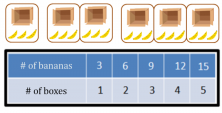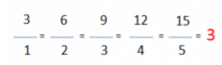Smartick is an online platform for children to master math in only 15 minutes a day

Jul13

# Direct Proportions: What Are They? What Are They Used For?

Today we are going to learn what direct proportions are and what they are used for.

Before we go on, we need to know what a magnitude is.

A magnitude is an amount that can be measured or counted. For example, the weight of a person, the number of construction workers working, the number of bananas, the amount of dog food a dog eats, the distance between two towns or the speed of a horse at a full gallop.

All of these can be related with other types of magnitudes.

### Direct Proportions:

• The weight of a person can be related to the clothing size that they might use.
• The number of construction workers that are working can be related to the how much time it takes to finish a project.
• The number of bananas can be related to the number of boxes needed to store them.
• The distance between two towns can be related to the time it takes in traveling between them.
• The speed of a galloping horse can be related to the time it takes a horse to reach one point from another.

There are various kinds of relationships. Today, we are going to look at one of them: direct proportions.

In order for two magnitudes to maintain a relationship that is a direct proportion, they have to be related in a way that if we double one, the other doubles as well. Likewise, if we triple one, the other magnitude needs to triple, too. It’s the same case for reducing, if we reduce one by a half, the other also needs to reduce by a half. So basically, if we raise the quantity of one, the other needs to do so “proportionally.” What relationship can we see between the number of bananas and the number of boxes that we need in order to store them?You can see that for however many more bananas we have, we need more boxes, right? These two magnitudes maintain a relationship that is directly proportional.

It’s important to know that the ratio between two directly proportional magnitudes is always constant. In our example that we have the ratio is 3.The relationships of direct proportions appear frequently in our everyday lives.

### Examples

Have you ever bought candy? How do you figure out the amount of money that you need to pay for the candy?

What can you guys tell me about these two magnitudes: the number of pigs and the number of haystacks that are needed to feed them?Can you say that they maintain a direct proportionality?

If you want to learn how to solve proportionality problems, remember that at Smartick you have lots more proportionality exercises and problems to practice with.